# How and why do companies use IRR and MIRR in their decision making?

How and why do companies use IRR and MIRR in their decision making?

This post was part of TyroCity discussion forum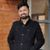Angel Paudel

IRR (Internal Rate of Return) is a breakeven point at which the NPV of the project equals to 0. While constructing the NPV profile of a project, it’s the point where the horizontal and vertical axis intersect (Martin, 1997). On the other hand, MIRR (Modified Internal Rate of Return) is also a similar concept but assumes that the cash flow the project generates over the time is actually reinvested at the cost of capital (Akpan, 1999). As most of the companies and startup do the same to amplify the rate of their growth, this is also considered to be more realistic compared to IRR.

Companies make use of IRR for various different reasons but one of it is that it makes the calculation of net return or profitability easier. As the rate of return is in percentage, the calculation and comparizations of the return (profit) along with the cost (expense) are easier to find. It makes the interpretation easier. Justifying this with an example, given a project has its cost of capital at 10% and IRR at 15%. From that, we can make out that the net return rate would be 5%. In any given case if the IRR is greater than the cost of capital the project is profitable. In the same scenario, if IRR was 5% instead of 15%, the project would have rather been at a 5% loss.

IRR is easy to calculate and even simpler for any stakeholder to understand. Given that I’ve no financial background before this, it wasn’t hard for me to grasp the concept and get on board. So, this easy to use feature added to it making easier to compare multiple projects in the selection process of a mutually exclusive project makes it more appealing. As the project with the highest IRR would be selected if there are two such projects and only one is to be selected. In case of selecting a project among mutually exclusive projects, let’s consider the company’s cost of capital to be 10%. Project A’s IRR value is 12% while project B’s IRR value is at 17%. So, this brings in the question, as both the project has IRR above the cost of capital, meaning both are to give a positive return to the business. In this case, the project with the highest IRR is to be selected, making the company in the decision making the stage of undertaking projects.

MIRR allows business to make changes to the stage by stage assumed rate of growth in a project. When a company makes an investment in a project, they make an investment to start the project (negative cash flow). As the project progresses over time, the company would start to make a return on the investment. This gives a positive cash flow to the business. When cash flow changes over the lifetime of the project within negative parameters or into the positive, one can observe multiple IRR for every change from positive to negative. The MIRR addresses this issue by accounting for both positive and negative cash flow separately (Mieila, 2017). Let’s take an example for this scenario, consider that a company makes an investment of NRs. 99,000 into a project, another company thought it would be interesting and funded NRs. 1,50,000 for the project. The main company again made an investment of NRs. 1,25,000 in the project. The project’s cash flow in this scenario moved from negative, positive and back to negative. The MIRR accounts for this change in cash flow while determining the return on investment. MIRR is also useful to a company in the decision making as it provides the company’s profit to be reinvested at a more realistic rate while also allowing the user to set the reinvestment rate. Companies can also make use of MIRR to compare projects with non-conventional cash flow methods to aid their decision making with regard to the selection of the project.

References

Akpan, E. (1999). A modified internal rate of return model for capital rationing in project selection. Production Planning & Control , 10 (8), 809-814.

Martin, R. (1997). Internal Rate Of Return Revisited. SSRN Electronic Journal , 1-3.

Mieila, M. (2017). Modified Internal Rate of Return. International Journal Of Sustainable Economies Management , 6 (4), 35-42.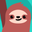Comment deleted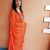Internal Rate of Return (IRR)

IRR estimates the discount rate that makes the value of subsequent net cash flows equal to the initial investment. It is the rate that makes the NPV of the project zero. In order to calculate the IRR, we need to consider the net present value of the project at two different discount rates. The IRR is calculated based on the assumption that the cash flows received during the project are reinvested at IRR (Gallo, 2016).

Using the formula,

IRR = LR + {NPV at LR / (NPV at LR - NPV at HR)} (HR - LR)

Where,

HR = Higher Rate of Return

LR = Lower Rate of Return

Example:

Let us consider a project with following cash flows and calculate its NPV at 15 % and 20 %.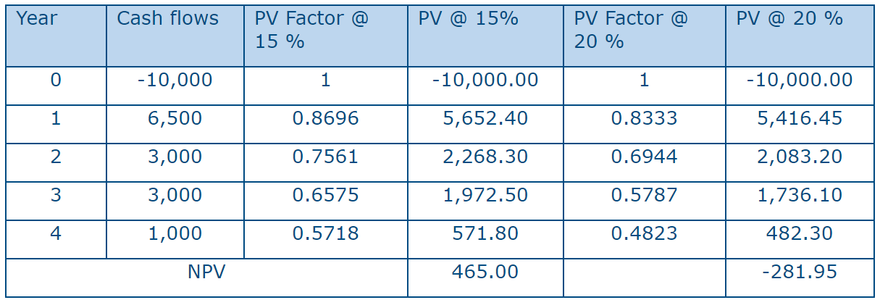Using the formula,

IRR = 15 + {465 / (465 + 281.95)} (20 - 15)
= 18.11%

The company uses IRR to find whether the project is worth investing or not based on the estimated percentage return offered by the project. If the calculated IRR is higher than the cost of capital, the investment is expected to increase the value of the firm and accepted. In the above example, if the cost of capital is less than 18.11 %, the project is accepted else it is rejected.

NPV and IRR both provide exactly the same decision regarding acceptance or rejection of the project in case of independent projects and projects with conventional cash flows. But in case of projects with unconventional cash flows and mutually exclusive projects with substantially different initial investments or substantially different timing of cash flows, NPV and IRR give contradictory results and make it difficult to take investment decisions. Similarly, sometimes there are multiple IRRs that increase the confusion (Moyer, McGuigan, Rao, & Kretlow, 2012).

Modified Internal Rate of Return (MIRR)

MIRR is the discount rate at which the present value of a project’s cost is equal to the present value of its terminal value. The advantage of MIRR over IRR is it assumes that cash flows from the project are re-invested at the at the cost of capital. Therefore it is better indicator of project’s profitability than IRR.

MIRR is calculated by solving this equation: Initial Investment = Terminal Value / (1 + MIRR) n

Example:

Let us consider the following two projects having cost of capital of 6 %.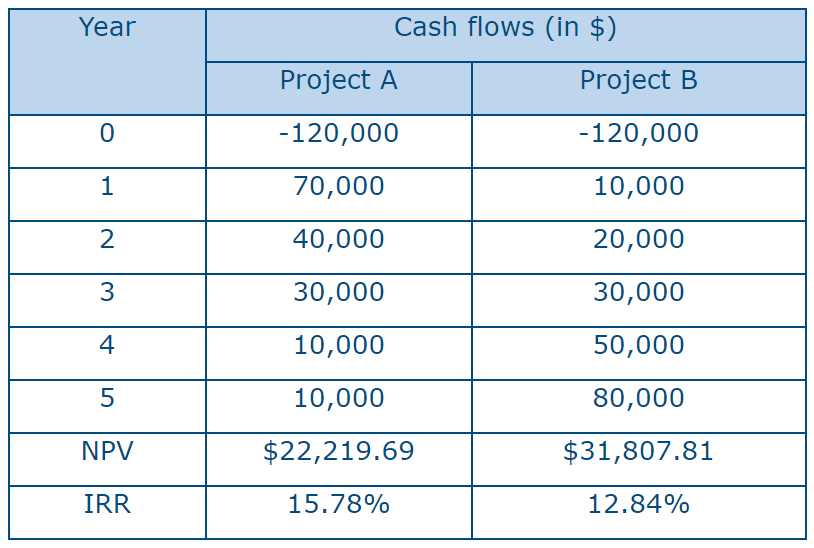In the above example, we can see that on the basis of NPV, project B is desirable and project A is desirable on the basis of IRR. In such situation, we calculate MIRR for these projects,

For Project A:

Terminal Value of Cash flow = 70,0001.064 + 40,0001.063 + 30,000 *1.062 + 10,000 * 1.061 + 10,000 * 1.060 = \$ 1,90,322.0272

Now,

1,20,000 (1+MIRR)n = 1,90,322.0272

or, MIRR = 1.58601/3 - 1

Thus, MIRR = 16.62 %

For Project B,

Terminal Value of Cash flow = 10,0001.064 + 20,0001.063 + 30,000 *1.062 + 50,000 * 1.061 + 80,000 * 1.060 = \$ 2,03,153.0896

Now,

1,20,000 (1+MIRR)n = 2,03,153.0896

or, MIRR = 1.69291/3 - 1

Thus, MIRR = 19.18 %

From the calculation, we can see that MIRR suggests Project B as better option for investment like NPV. Company uses MIRR because it gives a single correct answer for comparison of projects and avoid confusions. It is superior to regular IRR because it indicates the true expected long term rate of return (Ghimire, 2011).

References

Gallo, A. (2016, March 17). A Refresher on Internal Rate of Return. Harvard Business Review.

Ghimire, S. R. (2011). Fundamentals of Financial Management. Kathmandu: K.P Pustak Bhandar.

Moyer, R. C., McGuigan, J. R., Rao, R., & Kretlow, W. J. (2012). Contemporary Financial Management (12th ed.). Oklahoma: Cengage Learning.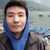Internal Rate of Return (IRR) is the discount rate used by companies that makes the NPV of projects equivalent to zero. It is an estimated rate so the actual rate of return may differ from this rate. This rate is just like the NPV. "Generally, the internal rate of return method indicates that a project whose internal rate of return is greater than or equal to the firm’s cost of capital should be accepted, whereas a project whose internal rate of return is less than the firm’s cost of capital should be rejected. (Moyer, McGuigan, Rao, & Kretlow, 2012)”

IRR also has some flaws that can make it difficult for the managers for selecting a project. Sometimes when a project has different cash flow (positive and negative) through the years or different scale of investments are made through the years, multiple IRR will be generated in one project itself. With multiple IRR the selection decision is different as we do not have any guidelines to choose which IRR to select.

It is in these circumstances where Modified Rate of Return is calculated. This helps calculate one rate and the decision for selection is taken after. For example let us calculate NPV in discounted rate of 0%, 4%, 8%, and 12% for 4 years.

Figure 1: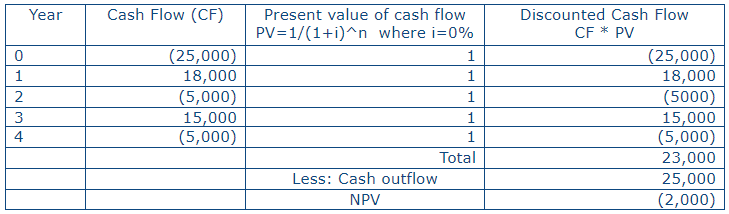Figure 2: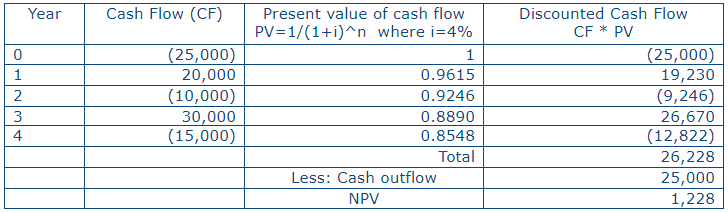Figure 3: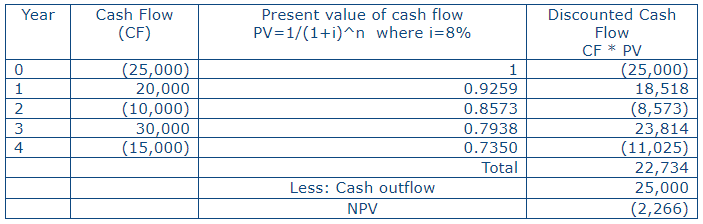Figure 4: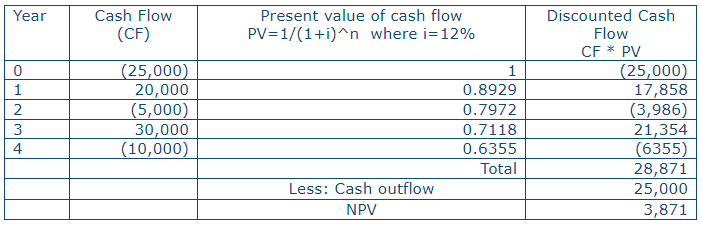Figure 5:These fluctuating NPV will generate numerous IRR in the X axis of the NPV profile. It is assumed that the cash flow generated in the project is reinvested but it is not clear as to what rate it should be reinvested in or if it is reinvested in the same project. Therefore for calculating the MIRR we calculate the future value of the cash flows and deduct it from the cash outlay to find the NPV. We then calculate the MIRR from this. The highest MIRR is accepted. MIRR is calculated as

MIRR = n Total Future Value of Cash flow/Cash Outlay

However MIRR is only used when there are multiple IRR. Its effectiveness in decision making is limited and so may not have financial significance.

Reference

Moyer, C. R., McGuigan, J. R., Rao, R., & Kretlow, W. J. (2012). Contemporary Financial Management. Natorp Boulevard: South-Western, Cengage Learning.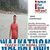How is Internal Rate of Return calculated?

The IRR should be greater than the given discount rate (cost of capital) to make a project acceptable. If IRR is less than the cost of capital then, the proposal cannot be accepted as it will lead to a negative NPV. Since, IRR is a rate of return, the project with a higher IRR is a rate of return, the project with a higher IRR should be ranked higher than the other project which has a lower IRR (Ross & Westerfield, 1996).

Why IRR is important?

• It considers cash flows of projects in their entirety.
• It takes into account time value of money.
• It is useful in ranking of projects because it is a rate and not any absolute value.
• It is independent of any externally determined rate (discount rate or cost of capital), and hence ranking of projects will not change with variation in cost of capital.
• It is particularly useful as it helps a businessman and also a financer in assessing the margin of safety in a project.
• It is more appealing to the businessmen who are used to thinking in terms of cost and return.

Drawbacks of IRR Methods

• The IRR method is based on the assumption that the recovered funds, if not consumed in each time period, are reinvested at i% rather than at MARR. This is not always practical.
• When the algebraic sum of the cash flow changes more than one in the series, it is possible to obtain multiple rates of return.

Let us consider the following cash flow

End of year Net Cash Flow
0 -1000
1 +2300
2 -1320

From the above cash flow pattern, we find IRR =10% and 20%, but actually both of them may not be correct. In such a situation, we may compel to abandon and the IRR and use Net Present Value criteria to make decision.

IRR method does not consider the scale of investment. Therefore, it can be misleading when choosing between mutually exclusive projects that have substantially different outlays.

For example,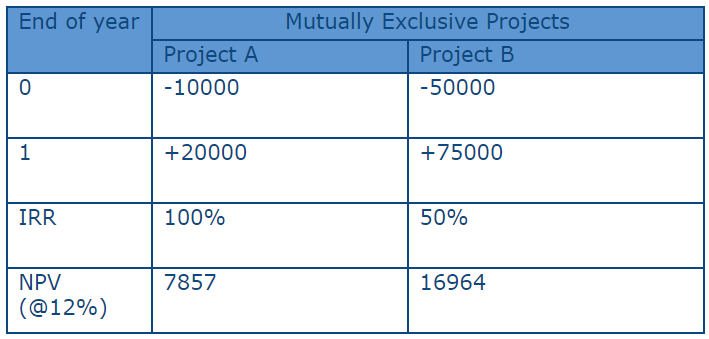Both projects are accepted at MARR=12% but project B with higher PW is more wrathful to the Stake holders, whereas from IRR point of view, project A seems better. Hence, IRR method is unsuitable for ranking projects of different scale of investment.

MODIFIED INTERNAL RATE OF RETURN (MIRR):

Why MIRR?

Internal rate of return is a good measure of profitability of a given project proposal. However, it suffers from an important drawback. It assumes that a project’s cash inflows are reinvested at the projects IRR. This may actually not be true. It is more reasonable to assume that a project’s cash flows are reinvested either at the cost of capital or any other rate at which opportunities of investment are available (which is usually lower than the IRR). With this assumption a modification in IRR is often suggested and this rate is called Modified Internal Rate of Return or MIRR; In this method we first calculate the terminal value of cash inflows at the cost of capital (or some other more realistic rate) and then discount it at a rate which will equalize its value with the present value of initial investment (Balyeat & Cagle, 2013).

How to calculate a MIRR of project:

Year CF
0 -1200
1 300
2 350
3 450
4 500

Assuming the cost of capital at 10%

The Modified Internal Rate of Return (MIRR) is calculated as follows:

Terminal values of inflows = 300(1.1)³ + 350(1.1)² + 450(1.1) + 500

= 1817.80

Discounted at 10%, its present value is 1241.58

Discounted at 11%, its present value is 1197.44

MIRR = 10 + (41.58) / (1241.58 - 1197.44)

= 10.94%

Decision: The MIRR is greater than cost of capital so that this project is Accepted.

Why MIRR is Important in capital budgeting?

• It does not need trial and error process to solve for i%
• There is no possibility of multiple rate of return.

References
Balyeat, R., & Cagle, J. (2013). Teaching MIRR to Improve Comprehension of Investment Performance Evaluation Techniques. JOURNAL OF ECONOMICS AND FINANCE EDUCATION ∙ Volume 12∙ Number 1 .

Ross, S., & Westerfield, R. (1996). Essentials of Corporate Finance. Irwin .Comment deleted